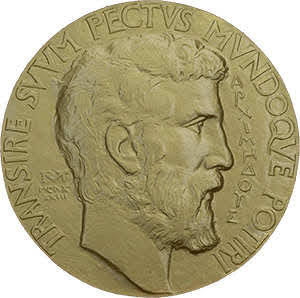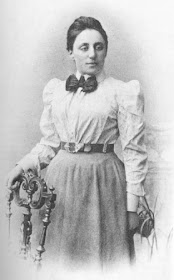### The first extragalactic black hole

In the world of black hole researchers, there is a group led by Tomer Shenar that, so far, has mostly demonstrated the non-existence of black holes previously announced by other teams.
As Shenar himself recalled, however,
For the first time, our group has come together to discuss the discovery of a black hole, instead of eliminating one.
We are talking about a black hole found inside the Tarantula Nebula, which is part of the Large Magellanic Cloud, one of the satellite galaxies of the Milky Way.
In particular, this black hole, of stellar mass, is of the "dormant" type, that is, it emits very low levels of X radiation, which are the radiations with which black holes are generally discovered.
This happens because the black hole interacts very little with its surroundings.
Another interesting aspect of the discovery is the absence of any trace of the star that generated the black hole.
[It] appears to have completely collapsed, with no sign of a previous explosion.
This black hole, the first extragalactic, was discovered orbiting a massive star thanks to six years of observations at ESO's Very Large Telescope.

### Fields Medals 2022I hope to write something about the Ising model in the next weeks, but in the meanwhile you can read something about E8 group. Below you can find the mathematicians that awarded the Field Medals 2022:
Hugo Duminil-Copin
For solving longstanding problems in the probabilistic theory of phase transitions in statistical physics, especially in dimensions three and four.
June Huh
For bringing the ideas of Hodge theory to combinatorics, the proof of the Dowling–Wilson conjecture for geometric lattices, the proof of the Heron–Rota–Welsh conjecture for matroids, the development of the theory of Lorentzian polynomials, and the proof of the strong Mason conjecture.
James Maynard
For contributions to analytic number theory, which have led to major advances in the understanding of the structure of prime numbers and in Diophantine approximation.
Maryna Viazovska
For the proof that the E8 lattice provides the densest packing of identical spheres in 8 dimensions, and further contributions to related extremal problems and interpolation problems in Fourier analysis.

### Noether's theoremThe Noether's theorem, discovered by German mathematician Emmy Noether, is one of the most sophisticated theorems in physics, a way to see how group theory, a branch of mathematics believed by many to be abstract, can provide the basis for an important physical concept. The premises of the theory of groups, coupled with the calculus of variations, lead to the conclusions of the theorem: the existence, under certain conditions, of conserved quantities within physical systems.
First of all we start with symmetry, one of the most important concepts for physics, and also the subject og group theory studies. To realize, therefore, this close link, it is enough to have in mind the statement of the theorem:
If a physical system exhibits some continuous symmetry, then there are corresponding observables whose values are constant over time.
A more sophisticated formulation of the theorem, on the other hand, goes something like this:
To every differentiable symmetry generated by local actions there corresponds a conserved current.
This more technical statement links the theorem and the symmetries with some of the most important groups for physics, the Lie groups. In the abstract of the Noether's paper, Invariant Variationsprobleme, in fact, we can read:
The problems in variation here concerned are such as to admit a continuous group (in Lie's sense); the conclusions that emerge for the corresponding differential equations find their more general expression in the theorems formulated in Section I and proved in the following sections.
The Noether's theorem, therefore, ensures that, when a physical system is invariant under the action of the transformations belonging to a Lie group, that is a group in which we are able to differentiate functions, then it certainly exists at least one conserved quantity, and this quantity and its invariance are expressed in the following equation: $\frac{\text{d}}{\text{d} t} \left ( \frac{\partial L}{\partial \dot x_k} \right ) = \frac{\text{d} p_k}{\text{d} t}$
Emmy Noether (1918). Invariante Variationsprobleme. de.wikisource.
English translation by Mort Tavel on arXiv

### Pi stories: Viète and the infinite seriesSpiked Math
Ludolph van Ceulen in 1596 using the polygon method, first came to calculate 20 decimal digits, then 35. Van Ceulen wasn't the last to use the method: for example Willebrord Snellius in 1621 calculated 34 digits, while the Austrian astronomer Christoph Grienberger in 1630 reached a record 38 digits using a 1040-sided polygon: this result is the most accurate ever achieved using the polygon method.
The infinite series supplanted this method: the first to use them in Europe was the French mathematician François Viète in 1593 $\frac2\pi = \frac{\sqrt2}2 \cdot \frac{\sqrt{2+\sqrt2}}2 \cdot \frac{\sqrt{2+\sqrt{2+\sqrt2}}}2 \cdots$ And in 1655 John Wallis $\frac{\pi}{2} = \frac{2}{1} \cdot \frac{2}{3} \cdot \frac{4}{3} \cdot \frac{4}{5} \cdot \frac{6}{5} \cdot \frac{6}{7} \cdot \frac{8}{7} \cdot \frac{8}{9} \cdots$ European mathematics, however, had come to this method only after Indian mathematics, albeit independently. In India, in fact, there is evidence of first approaches of this kind between 1400 and 1500. The first infinite series used to calculate $\pi$ is found, in fact, on the pages of the Tantrasamgraha (literally "compilation of systems") of the Indian astronomer Nilakantha Somayaji, circa 1500-1501. The series, presented without any proof (later published in the Yuktibhāṣā, circa 1530), was attributed by Nilakantha to the mathematician Madhava of Sangamagrama, who lived between 1350 and 1425 circa. Apparently Madhava discovered several infinite series, including many that contain the sine, cosine, and tangent. The Indian mathematician used these series to reach up to 11 digits around 1400, a value that was improved around 1430 by the Persian mathematician Jamshīd al-Kāshī using the polygon method.

### Mathematics and HIV

There is a link between mathematics and HIV that goes beyond the geometric structure of the virus, based on the icosahedron. Denise Kirschner describes this relationship very well in Using Mathematics to Understand HIV Immune Dynamics:
Since the early 1980s there has been a tremendous effort made in the mathematical modeling of the human immunodeficiency virus (HIV), the virus which causes AIDS (Acquired Immune Deficiency Syndrome). The approaches in this endeavor have been twofold; they can be separated into the epidemiology of AIDS as a disease and the immunology of HIV as a pathogen (a foreign substance detrimental to the body).(1)
The paper focuses on HIV immunology:
Our goal then is to better understand the interaction of HIV and the human immune system for the purpose of testing treatment strategies.(1)
The behavior of the immune system is schematized in this way:

### The entropy and the halting probability problem

The third law of thermodynamics states:
It is impossible for any procedure to lead to the isotherm $T = 0$ in a finite number of steps.
The theorem, discovered by Walther Nernst, is equal to say:
It is impossible for any process, no matter how idealized, to reduce the entropy of a system to its zero point value in a finite number of operations.
In classical thermodynamics we can define entropy, or the variation of entropy $\Delta S$, with the following equation: $\Delta S = \frac{\Delta Q}{T}$ where $\Delta Q$ is the heat's variation and $T$ is the temperature.

### The Berry's phase and the black hole

In quantum mechanics a geometric phase, also called Berry phase, is a phase difference that a given physical system acquires during a cycle in which the system itself is under the action of an adiabatic process. This phase is linked to the geometric properties of the system itself (which is a simplification, but for our purposes there is no need to go into too much detail).
It was discovered independently by Shivaramakrishnan Pancharatnam in 1956(1), Hugh Christopher Longuet-Higgins(2) in 1958 and subsequently generalized by Michael Berry(3) in 1984. This phase, although geometric, has measurable physical effects, for example in an interference experiment. An example of a geometric phase is Foucault's pendulum.
The most famous version of this experiment, designed by Léon Foucault, dates back to 1851 when the French physicist, with the aim of showing the rotation of the Earth around its axis, suspended a ball of 28 kilograms of lead coated with brass over a surface of sand using a 67 meter cable hooked to the top of the dome of the Panthéon in Paris. The plane of the pendulum was observed to rotate clockwise at approximately 11.3 degrees per hour, completing a full circle in 31.8 hours. A more refined examination shows that after 24 hours there is a difference between the initial and final orientation of the trace left on Earth which is equal to